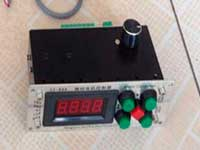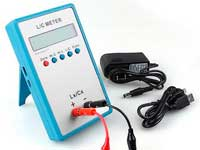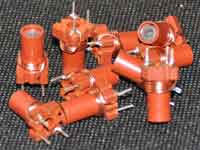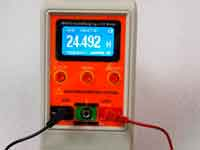Calculation of inductance multiturn round bundle loop with round cross-section (for metal detectors). - 3.5 out of 5 based on 2 votes
Rating 3.50 (2 Votes)

Calculation of inductance multiturn round bundle loop with round cross-section (for metal detectors).Plugin multiloop calculates the coil in the form of a round multi-turn wiring bundle with round cross-section. Such coils are often used as induction sensors for metal detectors. This coil is an outstanding representative of short coils and this coil cannot be calculated by the empirical Wheeler's formula for multilayer coil.
What is the way out? Firstly, you can apply a numerical method of calculation according to Maxwell's formula. However, this method is quite bulky for the coil with a circular cross-section of the wire bundle. If the coil has a square cross-section, it can be calculated as a multilayer inductor directly in the program Coil32. Note that in the Coil64 app and in the Coil32 for Android the search coil for a metal detector calculates by the Brooks formula.

To calculate the round multiturn wire harness ring, the plug-in multiloop uses the following empirical expression:where:

• L - inductance (H)
• N - number of turns of the bundle
• d - average diameter of the bundle (m)
• Φ - auxiliary factor, calculated according to the formulawhere: γ = r/d

The numerical calculation method is the "virtual adding" of turns until the required inductance will be reached. The resulting diameter of the bundle is calculated by the following formula:where:

• dw - wire diameter
• n - the number of turns of the loop, rounded to the nearest larger integer.

In the case of a single turn the diameter of the bundle is equal to the diameter of the wire.

P.S: An average diameter of the bundle isn't the input parameter in the plug-in, the input parameter is the inner diameter of the ring.Сomments from anonymous guests are enabled with moderation.

Coil Winding MachineUS \$187.53
The system consists of individual components including a controller, a stepping motor, a foot switch, power adapter and output shaft. This design makes it very easy for you to integrate the system into your own project.

LC Meter LC200AUS \$37.99
Measuring Capacitance Range:
0.01 pF - 100mF
Measuring Inductance Range:
0.001 uH - 100H
Measurement accuracy:1%.

RF InductorsUS \$6.43
Variable Ferrite Core Inductor coil set 3.5Turns 25-100MHZ

Auto Ranging LCR MeterUS \$63.07
Meter can measure 0.00pF to 100.00mF capacitance, 0.000uH to 100.00H inductance and 0.00? to 10.000M? resistance. Very suitable for small value capacitor and inductor measurement.